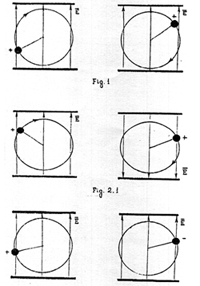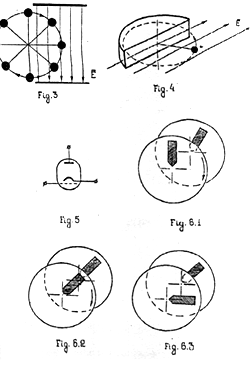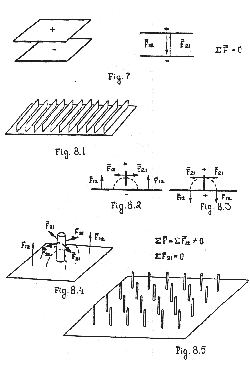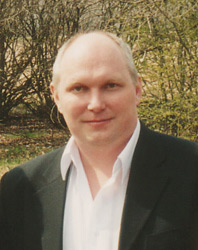Potentials in power generation
Alexander V. Frolov

This paper was published in Newsletter of Planetary Association for Clean Energy, Vol.8(1), Sept.1994. It is the same paper that was published at first in New Energy News, May 1994, but in this version second part here is edited by Thomas E. Bearden, Association of Distinguished American Scientists. His address: POB 1472, Huntsville, Alabama 35807, fax (205)536-0411

Professional physicists dislike considering the use of potentials for the creation of work in the load.
This is explainable in view of their staunch footings in the Law of Conservation of Energy.

Yet it is possible to demonstrate there is in fact no rule in Nature against free energetics. The simple
availability of potential fields is a sufficient condition for the driving of fuel-less power systems.
Once such a system is operational, certain effects emerge which are connected to the Law of Conservation of Energy and which suggest the formulation of this Law in a more general view.

The solution of the reactionless support-free motion problem is provided by means of asymmetrical potential interaction is also proposed.

Total work of the potential field

There is an "indisputable" formulations that is universally applied by physicists: the total work of the
Potential field along a closed trajectory is equal to zero. Indeed, from Fig.1  we can see that part
of the work is positive (acceleration) and the other part ot the work is negative (deceleration).Fig. 1, Fig.2.1, Fig.2.2.

In Physics textbooks, the task of applying the Potential for making only positive work in both
parts is absent. This may be explained by the unwillingness of textbooks writers to discuss the
more interesting question of energy transformations when the inner structure of space-time is
changed. Figure2 shows the same charged mass M rotation in an potential electric field. The difference is in the mode of interaction. The polarity of it is changed when positive work is changed to negative. Figure 2.1 shows that the direction of field is changed. Figure 22. shows that the sign of the charge is changed.

There is a another set of simple examples of application of potential field for the creation of only
positive work in the load. Figure 3 is an arrangement similar of a water mill wheel.Fig.3, Fig.4, Fig.5, Fig.6
Now let's compare this to Figure 1. Can you see the miller who submerged the entire wheel in the river water flow and thereby 'scientifically' proved that it is impossible to use water flow for the creation of power ? But our professors use a similar argument to show that the total work of the potential in a closed cycle is always zero !

Actually, the part of the trajectory, or periodic cycle which constitutes negative work (deceleration) portion may be excluded by means of spatial superimposition, pulsed mode, change of interaction polarity, or by means of a screen (Fig. 4.)

Fig. 5 shows that changes in the arrangement of electrodes ot vacuum tubes allow one to increased the energy of emission current electrons.

These basic schemes concepts require further development for industrial application, of course.
Note that Coulomb's interaction is inversely proportional to the square of the distance, so it is
necessary to try to decrease this distance to obtain greater interaction. Figure 6 shows a "free energy"
device similar to a well-known electric machine. Let us consider the interaction of 2 segments that
have opposite charges. In the case of a constant sign of interaction, the process corresponds to the
above "totally submerged" water wheel conclusion of the miller. Figure 6. 1 shows that the attraction
between segments creates a propulsion force and that the rotor is moving with some acceleration.
Figure 6.2 notes the point of balance, and then Figure 6.3 shows that the rotor is decelerated
since segments remain in attraction. The total work of the potential field is equal to zero. But why?
It is not an indispensable condition of Nature. It enough to change the sign of interaction between
segments, after the balance point, from attraction to repulsion to create the mode of acceleration
again!  And this can be done by judiciously switching (reversing) the potential itself at the appropriate time. In this case both halves of the cycle are in acceleration. We omit the technological and descriptions of the group of contacts, collector brushes, condensers, transformers, and so on.

It is not news that potential fields can perform work Any textbook show examples of  closed
circuit  negative-positive cycling of such work and proves that the total work of successive acceleration and deceleration is equal to zero. But since this closed circuit process is actually the successive performance of two work processes, it is possible to change the parameters of one process and change the cycle into the sum of two or several sequential parts, each of positive work The trick is in the point-momentum of balance.

The asymmetrical potential interaction

Let us consider the use of potential field for the creation of propulsion force and reactionless, support-free motion. Figure 7 shows an ordinary flat electric condenser.Two flat plates are in attraction (different charges) or in repulsion (like charges). The total resultant force of the two-plate system equals to zero and the material system of the charged condenser remains immobile. To discover a solution for a non-zero unilateral resultant force, all we need is to note that the force line of the potential field is always perpendicular to the charged surface and take advantage of that fact.

Figure 8 shows the same condenser but one plate is perpendicular with regards to the other plate.
The vertical plate is divided into several elements in order to decrease distance of Coulomb's interaction between plates. The total force resultant between any two vertical elements is the sum of
opposing forces and it equals to zero. But the total force that applies between a vertical plate and the
horizontal plate is a sum of co-directional elementary forces and it is not equal to zero. The system
thus moves by means of its asymmetrical potential interaction.

Note that the perpendicular elements may have cylindrical shape. The propulsion force may be
regulated by means of change of angle between plates of the asymmetrical condenser or by of a
changing the potential.

The well-known experiments with "inertioidal" moving systems may be considered as a particular
case of asymmetrical (vector) potential technology. When eccentric mass is moving along epicycloidal or cardioidal trajectory, it creates a propulsion force that is the result of the gradient of
acceleration . This process is described by a vector potential conception. Since asymmetrical scalar potential interaction does not require additional power after is generation, the scalar technology is
more interesting for the aerospace industry than inertial propulsion systems.

(With T.E.Bearden's  commentary)

We pose a common sense question: Where do we take the power from, for making work in the load, if we use potential energy for it and the potential is not exhausted? Note that the existence of force effects of potential fields must raise the same question.

Why magnets don't exhaust?

Why can a simple permanent magnet (with its vector potential) hold and suspend a massive
piece of metal when in so doing it continually makes work against force af gravity and yet it does
not require any fuel and power And the magnet does not weaken and is not exhausted?

E. T. Whittaker's conception of potential as a bi-directional flow of energy is developed by T.E.
Bearden in Gravitobiology. The generalization of Newton's Third Law in this conception leads to a
requirement of complementary pairs of conjugate photons for the electromagnetic radiation process.
Since the process means the change of information (i.e., of the structure of space) in time, the
pair's anti-process takes place in reverse-time. Certainly, the anti-process is developed from its
own past time in the future, but from our point of view it is changes from the future in past time. In
this generalized Newton's Third Law the inner structure of the electric potential field is created by
two oncoming flows of energy: the photons are spreading from the charge-source of the potential
field and the antiphotons are inflowing into that point-centre of charged mass.
Now let's return to a question that rings true: Since "something" cannot spring up from "nothing", what is "reduced' or depleted, and where, if in the local area of our space-time the free energy process takes place and some power is dispersed in the load?

T. D. Lee's "vacuum engineering"

Let's generalize this question. 'Space-time" and "potential" and "energy" are identically the same
thing. In this conception, the well-known ability of energy to change its form is extended to allow
energy to "change" into a space-time change, and vice-versa. This notion is already inherent in general relativity. In this manner we invert the usual notion that energy moves through an inert, featureless space-time (vacuum) to interact upon a mass and alter its energy, position, mass, charge.
or all four. Instead, we now have extended and applied John Archlbald Wheeler's admonition
that "Space acts on matter, telling it how to move" .  Bearden calls such action upon a mass by the local space-time and its dynamic structure a vacuum engine, after the "vacuum engineering" suggested by Nobelian T. D. Lee. 

Thus from "energy" as "ordering and disordering" of the hidden spatial structure, we arrive at energy
as a descriptive of any process. The note of "process" as the change of information or spatial structure in time from the point of view of the observer explains the effects that are connected with
changes of natural potential field energetics balance.

Potentials are bi-directional

So the potential field can be considered as a bi-directional process/anti-process. Usually energyinformation that is radiated by the source of the potential field (the direct process in time) has some difference with the energyinformation that is received (the anti-process in reverse-time). This difference causes the rate of time for this mass object and causes the existence of this mass as the
source of the potential field. This difference is equal to trapped energy of mass-form that can be
transformed into radiation energy, as the cause of mass decay. If the potential field is used only for
making positive work of acceleration, then according to the Conservation Law the power process in
space-time must be balanced by a corresponding power anti-process in the space of reverse-time.
This imbalance of bi-directional flow of potential energy shows itself as a local change of spatial
curvature and a change in the local rate of flow of time.

High energetic vacuum

The concept of the highly energetic Vacuum is fully accepted in physics. It has been thermodynamically shown that in principle the electrical energy of the vacuum can be extracted as heat and power . It only remains to make the local vacuum potential partially asymmetrical, then gate and freely utilize the energy available from that asymmetry.

If it is necessary for technology, it is possible to make calculations of the amount of power that
must be dispersed as heat to obtain the mode of total compensation of natural spatial planet curvature and rate of time in the local area of space-time.

The comprehensive conservation

So it is impossible to create "something" from "nothing". But the creation of an external "free energy" flow process is balanced by a corresponding internal anti-process and you do not create      "something from nothing" from the point of view of Eternity. Consequently such a process does not
violate the laws of physics.

Further, mass itself consists of trapped energy, so it may be considered to be a very strong, localized
scalar potential . This potential also must consist of bi-directional wave pairs, and thus be subject to direct electromagnetic engineering by means of external alteration of its constituent Whittaker waves.

The unreality of matter

So after Whittaker's representation of conventional electromagnetics as a function of two scalar
potentials and his decomposition of any potential into bi-directional wave pairs, it becomes possible
experimentally verify the old philosophical idea of the unreality of material existence. The departure from the static notion of mass to a dynamic description of the material world's existence allows the development ot the conception of the electromagnetic design template of local physical reality. Since the mass (and matter) is a process that is balanced by a reverse-process, there are no difficulties in principle to develop such technologies as transmutation of chemical elements , materialization and dematerialization, teleportation and so on.

No violation at Law of Conservation

The application of potential energy for the creation of power does not violate the Law of Conservation of Energy that remains true in the more general global view:

The complete energy of a four-dimensional system is the sum of its energy of space-time processes and its energy of space-reverse-time processes, and it is constant and equal to zero.

References

1. W. Misner, K.S. Thorne and J.A.Wheeler, Gravitation, W.H. Freeman and Co., San Francisco, 1973, p.5.
2. Lee, T.D. Particle physics and introduction to fled theory. Harwood. New York, 1981. Ch. 25: Outlook: Possibility of vacuum engineering: p. 824-828.
3. Cole, Daniel C. and Harold E. Puthoff, Extracting energy and heat from the vacuum, Physical Review, Vol. 48, No. 2, Aug. 1993, p.1562-1585.
4. T.E. Bearden, A redefinition of the Energy Ansatz, leading to a fundamentally new class of nuclear reactions. Proceedings of the 27th Intersociety Energy Conversion Engineering Conference (IECEC 92). Town & Country Hotel Convention Centre, San Diego, California, 1992, p. 4.303-4.310.

T.E.Bearden

I am grateful to Dr. Bearden for value editions and references on my paper.a2509@yahoo.com